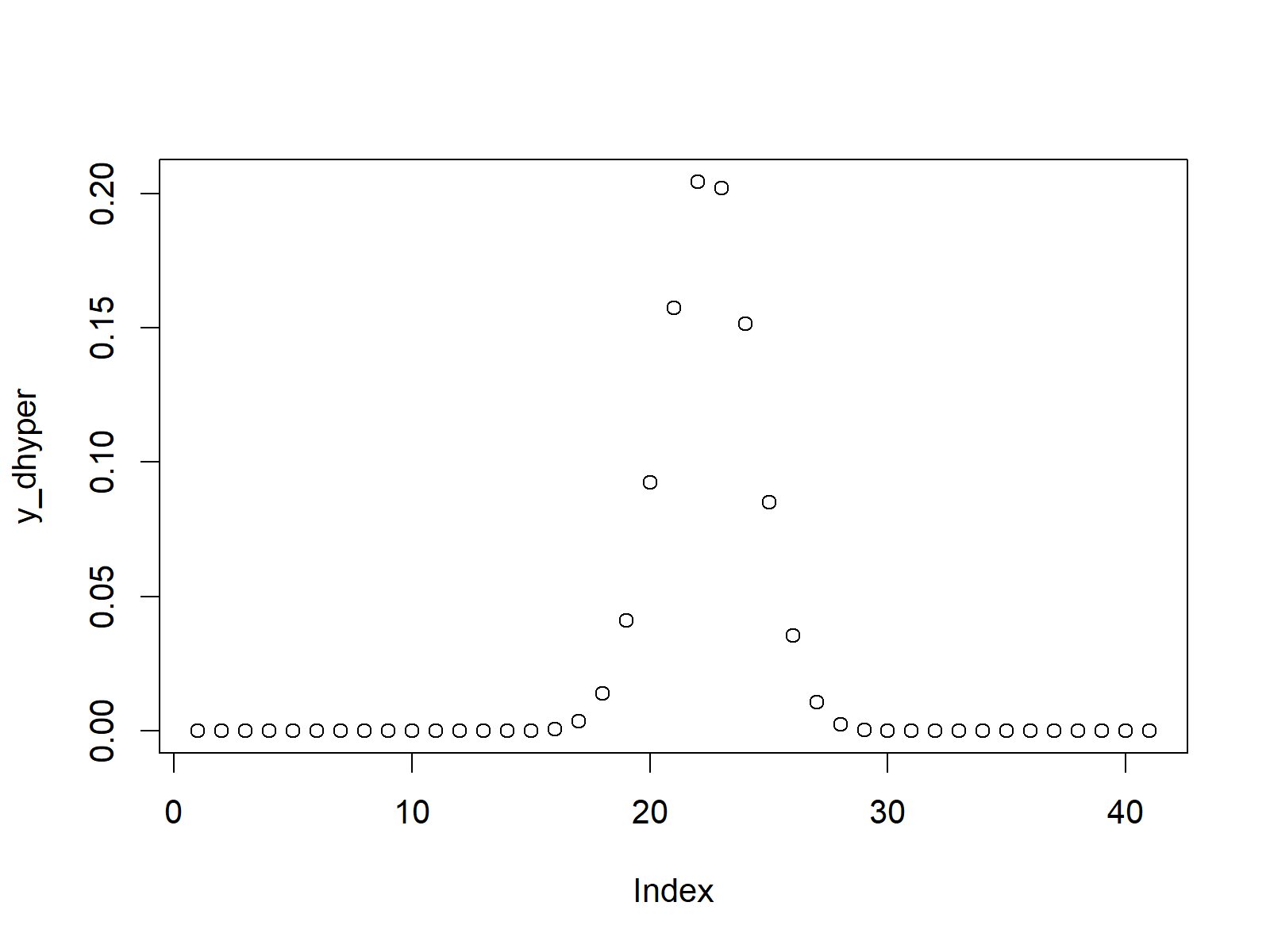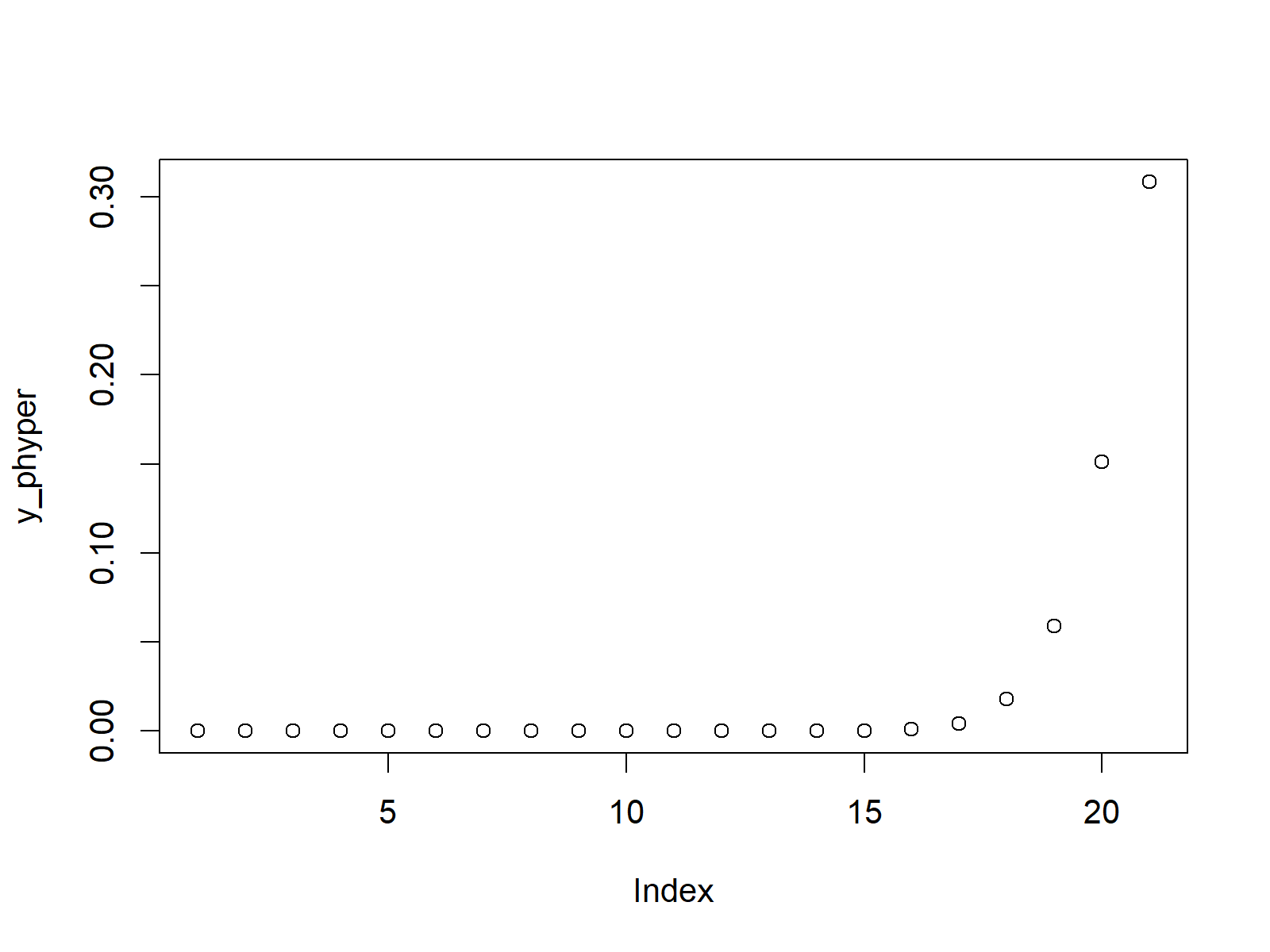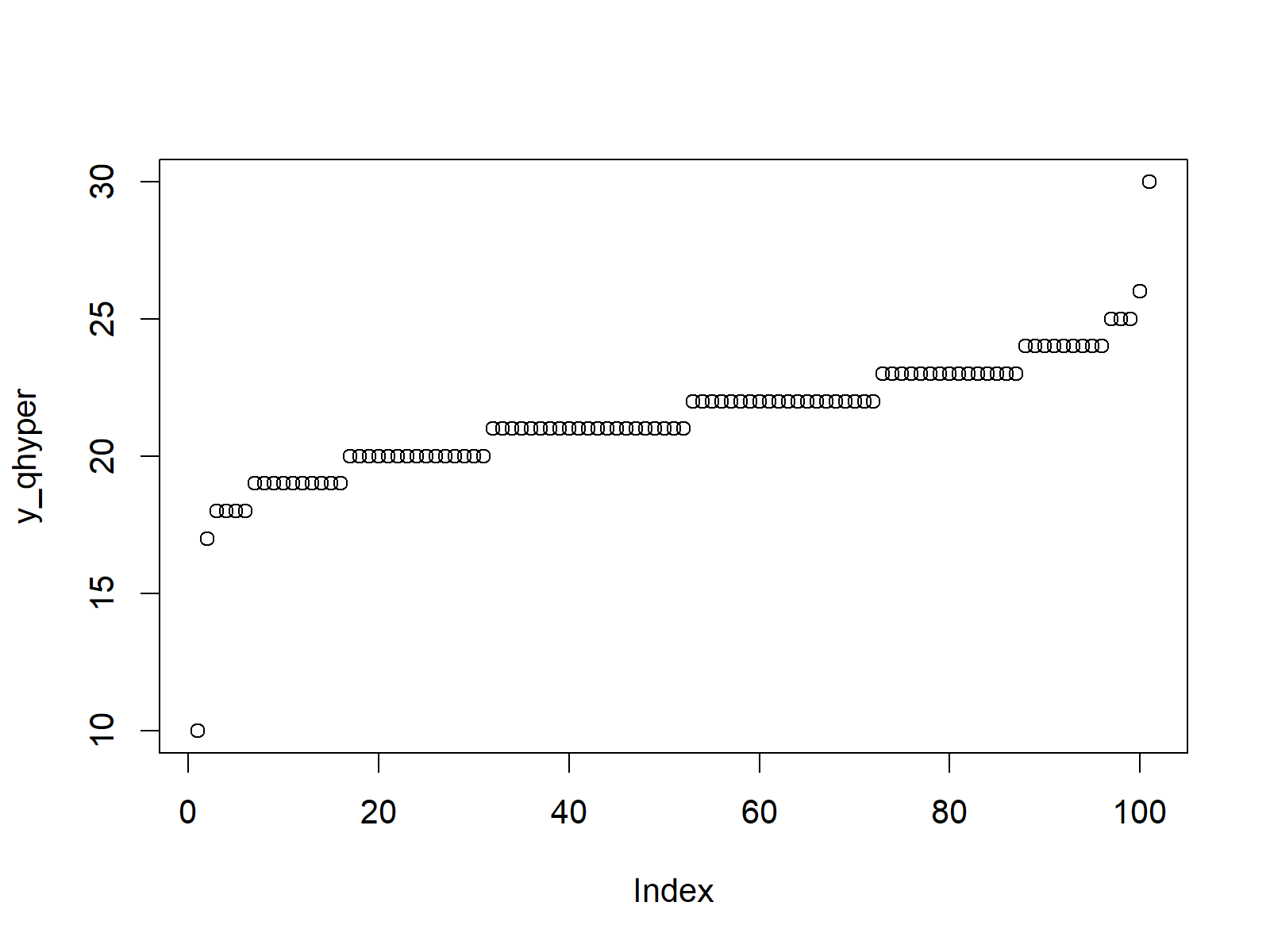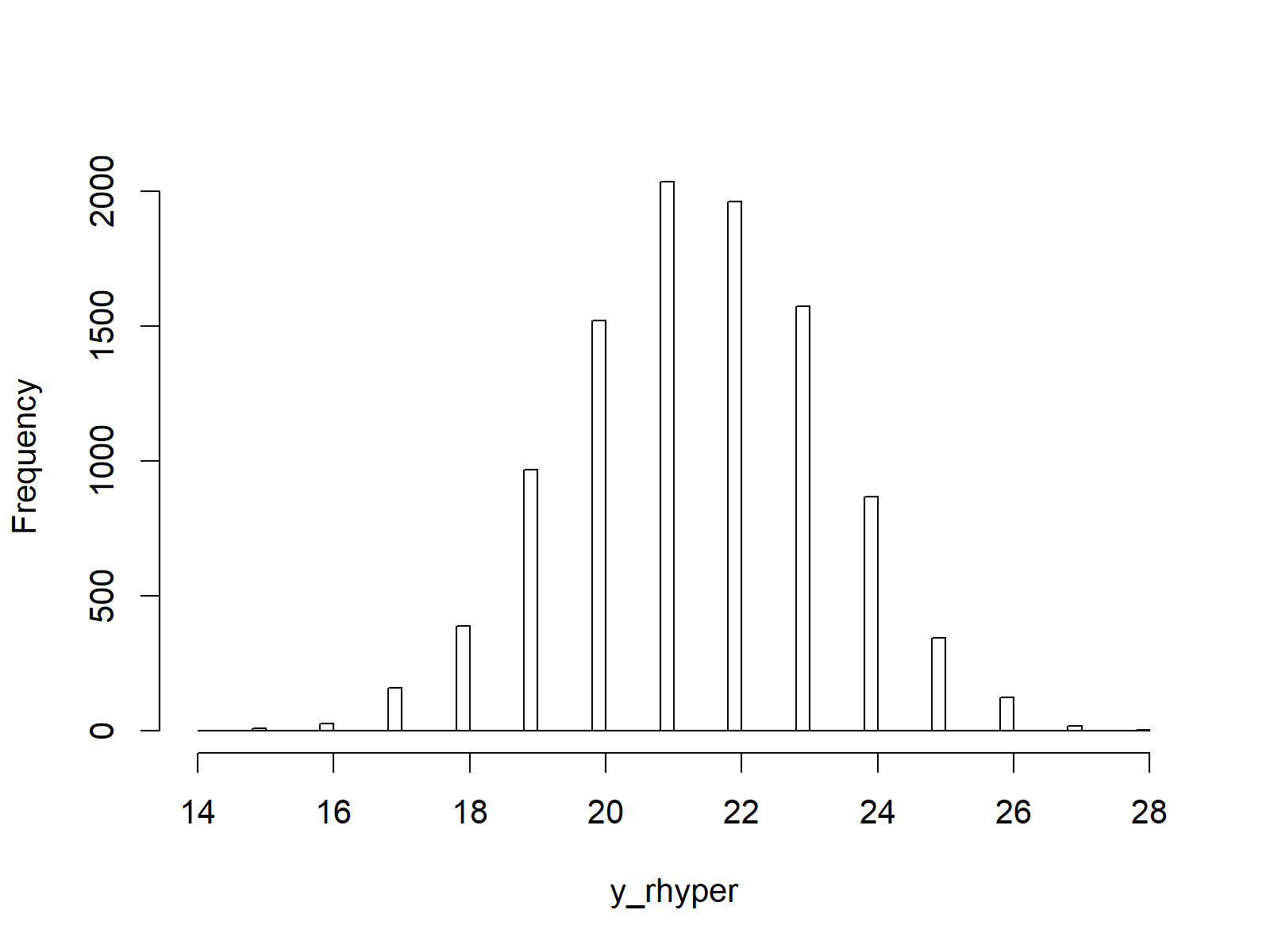# Hypergeometric Distribution in R (4 Examples) | dhyper, phyper, qhyper & rhyper Functions

This article explains how to use the hypergeometric functions in the R programming language.

The post is structured as follows:

Let’s start right away:

## Example 1: Hypergeometric Density in R (dhyper Function)

Let’s start in the first example with the density of the hypergeometric distribution. To get the density values, we need to create a vector of quantiles:

`x_dhyper <- seq(0, 40, by = 1)                          # Specify x-values for dhyper function`

Now, we can apply the dhyper R command to this vector of quantiles.

In order to apply the dhyper function, we also need to specify the parameters m (i.e. the number of white balls in the urn), n (i.e. the number of black balls in the urn), and k (i.e. the number of balls drawn from the urn). I set these parameters to 50, 20, and 30. However, you might modify these parameters to your personal preferences.

`y_dhyper <- dhyper(x_dhyper, m = 50, n = 20, k = 30)    # Apply dhyper function`

After applying the dhyper function, we can create a graphic representing the output of the dhyper function:

`plot(y_dhyper)                                          # Plot dhyper values`Figure 1: Hypergeometric Density.

## Example 2: Hypergeometric Cumulative Distribution Function (phyper Function)

The second example shows how to produce the hypergeometric cumulative distribution function (CDF) in R. Similar to Example 1, we first need to create an input vector of quantiles…

`x_phyper <- seq(0, 20, by = 1)                          # Specify x-values for phyper function`

…then we can apply the phyper function to this vector…

`y_phyper <- phyper(x_phyper, m = 50, n = 20, k = 30)                # Apply phyper function`

…and finally we can produce a plot representing the hypergeometric CDF:

`plot(y_phyper)                                          # Plot phyper values`Figure 2: Hypergeometric Cumulative Distribution Function.

## Example 3: Hypergeometric Quantile Function (qhyper Function)

Example 3 illustrates the R code for the hypergeometric quantile function. First, we have to specify a sequence of probabilities between 0 and 1:

`x_qhyper <- seq(0, 1, by = 0.01)                        # Specify x-values for qhyper function`

Then, we can apply the qhyper function:

`y_qhyper <- qhyper(x_qhyper, m = 50, n = 20, k = 30)    # Apply qhyper function`

And finally, we can produce a plot representing the hypergeometric quantile function:

`plot(y_qhyper)                                          # Plot qhyper values`Figure 3: Hypergeometric Quantile Function.

## Example 4: Generating Random Numbers (rhyper Function)

If we want to produce a set of random numbers distributed according to the hypergeometric distribution, we need to specify a seed and a sample size that we want to simulate first:

```set.seed(53535)                                         # Set seed for reproducibility
N <- 10000                                              # Specify sample size```

Now, we can apply the rhyper function to generate our random numbers:

```y_rhyper <- rhyper(N, m = 50, n = 20, k = 30)           # Draw N hypergeometrically distributed values
y_rhyper                                                # Print values to RStudio console
# 22 22 20 20 20 21 24 21 22 24 22 23 20 20 22 24 21 22 25```

The following histogram shows the distribution of our random numbers (i.e. a hypergeometric distribution):

```hist(y_rhyper,                                          # Plot of randomly drawn hyper density
breaks = 70,
main = "")```Figure 4: Hypergeometric Random Numbers.

## Video & Further Resources

Have a look at the following video of my YouTube channel. I’m explaining the R codes of this article in the video.

You could also have a look at the other tutorials on distributions and the generation of random numbers in R:

Furthermore, you may read the related articles on this homepage.